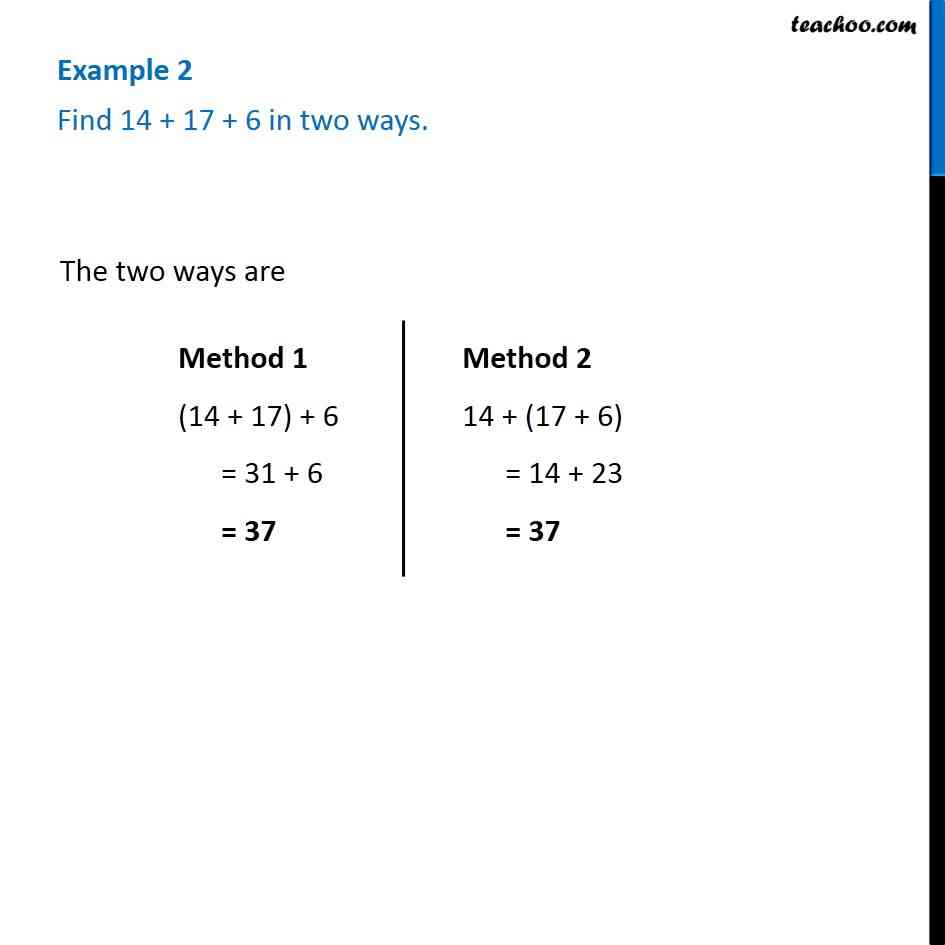Practice Questions

Chapter 2 Class 6 Whole Numbers
Serial order wiseLearn in your speed, with individual attention - Teachoo Maths 1-on-1 Class

### Transcript

Question 2 - Chapter 2 Class 6 Whole Numbers - NCERT Book Find 14 + 17 + 6 in two ways. The two ways are Method 1 (14 + 17) + 6 = 31 + 6 = 37 Method 2 14 + (17 + 6) = 14 + 23 = 37